## Simple Harmonic Motion Quadratic Perturbation

Given a simple harmonic oscillator with a quadratic perturbation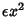,(1)

find the first-order solution using a perturbation method. Write(2)

so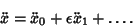(3)

Plugging (2) and (3) back into (1) gives(4)

Keeping only terms of orderand lower and grouping, we obtain(5)

Since this equation must hold for all Powers of, we can separate it into the two differential equations(6)(7)

The solution to (6) is just(8)

Setting our clock so thatgives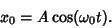(9)

Plugging this into (7) then gives(10)

The two homogeneous solutions to (10) are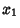(11)(12)

The particular solution to (10) is therefore given by(13)

where(14)

and the Wronskian is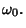(15)

Plugging everything into (13),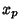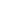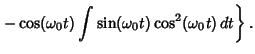(16)

Now let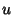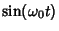(17)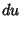(18)(19)(20)

Then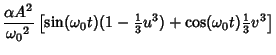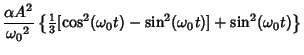(21)

Pluggingand (21) into (2), we obtain the solution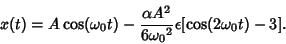(22)